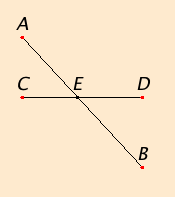# Proposition 15

If two straight lines cut one another, then they make the vertical angles equal to one another.

Let the straight lines AB and CD cut one another at the point E.

I say that the angle CEA equals the angle DEB, and the angle BEC equals the angle AED.I.13

Since the straight line AE stands on the straight line CD making the angles CEA and AED, therefore the sum of the angles CEA and AED equals two right angles.

I.13

Again, since the straight line DE stands on the straight line AB making the angles AED and DEB, therefore the sum of the angles AED and DEB equals two right angles.

But the sum of the angles CEA and AED was also proved equal to two right angles, therefore the sum of the angles CEA and AED equals the sum of the angles AED and DEB. Subtract the angle AED from each. Then the remaining angle CEA equals the remaining angle DEB.

Similarly it can be proved that the angles BEC and AED are also equal.

Therefore if two straight lines cut one another, then they make the vertical angles equal to one another.

Q.E.D.

### Corollary

From this it is manifest that, if two straight lines cut one another, then they make the angles at the point of section equal to four right angles.

## Guide

Although the term vertical angles is not defined in the list of definitions at the beginning of Book I, its meaning is clear form its use in this proposition. Vertical angles are angles across a vertex that share lines as their sides.

A corollary that follows a proposition is a statement that immediately follows from the proposition or the proof in the proposition. It is possible that this and the other corollaries in the Elements are interpolations inserted after Euclid wrote the Elements. During the writing, he could have either bundled the corollary into the proposition or made it a separate proposition.

### Proclus’s Corollary

Proclus (412–485) includes another corollary: If any number of straight lines intersect one another at one point, then the sum of all the angles so formed equals four right angles.

#### Use of Proposition 15

This proposition is used in the next one, a few others in this book, II.10, IV.15Clark University